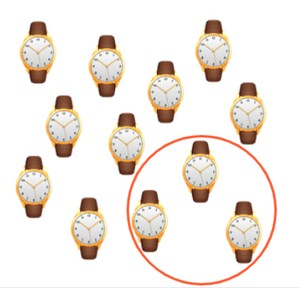Calculating fractions as part of a whole

# Calculating fractions as part of a whole

I can calculate simple fractions as part of a set.8,000 schools use Gynzy92,000 teachers use Gynzy1,600,000 students use Gynzy

## General

In this lesson, students will learn the steps (using division) to calculate simple fractions as part of a set.

## Standards

CCSS.MATH.CONTENT.3.NF.A.1

## Learning objective

Students will be able to calculate simple fractions as part of a set.

## Introduction

Students will look at pictures and decide what fraction they see. Knowing how to find fractions will help students know how many objects you get if you are given the fraction.

## Instruction

A fraction has a numerator and denominator. A character will model the steps to calculate fractions with a sample problem: find the fraction of the set you want to know and divide the total by the denominator. Students will then practice this skill by calculating one-third of a group of marbles. They will do a few other problems using simple division to calculate one-half and one-fifth. They will also do word problems.

## Quiz

Students will complete 10 questions.

## Closing

The lesson closes with 3 more practice problems.

### The online teaching platform for interactive whiteboards and displays in schools

• Save time building lessons

• Manage the classroom more efficiently

• Increase student engagement